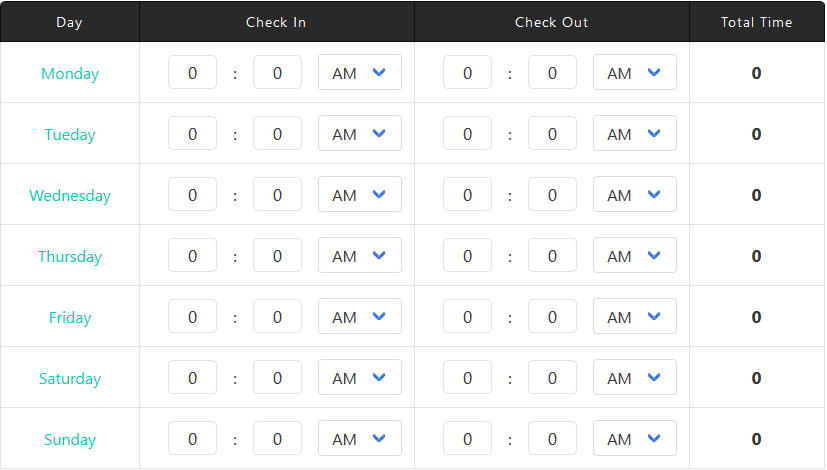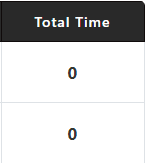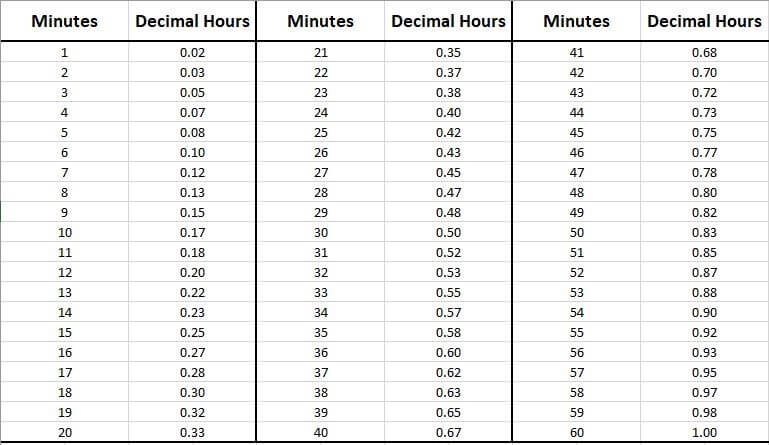# Time Card Calculator: Daily and Weekly Timesheet

This Timecard Calculator will easily allow you to calculate your working time or overtime. The primary use for this one is to compute the hours you spent working each day in a week. What makes it more fun is that you can easily print or email the results to your boss with a single click.

1. You can Enter the timings from Monday to Sunday.
2. Use IN Box to Enter the time of work starting.
3. Use OUT Box to Enter time of leaving.
4. Use Dropdown Button for selecting AM/PM
5. You will get Total Time of each day in the blue text box.
6. See the Grand Total Box for all days results.
7. Use Clear Button to Clean the entire sheet written.
8. Use Print Button To Print the complete TimeCard.
9. Use Email Box if you want to recieve the complete TimeCard at your Email ID.
Day Check In Check Out Total Time
{{timeSlot.day}} Check In
:
Check Out
:
Total TimeAbout the Author Nauman Rehmat Nauman is a Digital Marketing Specialist and owner of several online tools like DrEmployee. He believes in helping common people by providing a free online solution to day to day tasks. This project is one of them to offer free financial tools and tips.

## How to Use Time Card/sheet Calculator?

Deducing the total hours worked in a week and weekly wage from a Timecard can be a precarious job. You have to process your work Time In and Time Out for a whole week, followed by the calculations of time durations, and then convert them into 24 hours format, which is finally added up to give total hours worked in a week.

During this whole process, a single mistake can have a significant impact on results. Consequently, you will end up calculating your wrong salary.

To prevents such blunders, you can use our Timesheet Calculator, which features a user-friendly interface with 100% accuracy, giving you instant results.Here is the Step by Step Guide to using our Timecard Calculator:

Step # 1:Once you have launched the Time Card Calculator, you will see a table with textboxes for Check-In and Check-Out time for each day.

Enter your Hours and Minutes for Check-In and Check-Out of Work on their respective text boxes for each day.

Step # 2:Beside every Textbox of Time In and Time Out, there is a drop-down menu to choose between AM and PM. Use them accordingly.

Note: You will get the Total Time duration of work for each day in the last column.

Step # 3:Once you have entered Check-In and Check-Out time of at least one day, You would start to see your Grand Total of total hours worked in a week at the bottom of the table.

Enter all the details by following the steps above to get your accurate Grand Total of Total Hours worked in a Week instantly.

Step # 4:• You can use the red CLEAR button to reset the calculator.
• You can use the PRINT button to print the entire sheet with the result.
• You can also email the sheet by entering the recipient's Email address in Your Email textbox and pressing Email

## FAQs:

1. To calculate time on Time Card (1 week) follow the following steps:
2. Convert all you Time In and Time out on Time Card into 24-Hours Format (Military Time). You can also use our Military Time Converter to convert instantly and accurately.
3. Now subtract each Time In from Time Out to determine the time duration of work of each day.

Note: Subtracting TWO times isn't the same as subtracting two numerical values. It's a bit tricky for which you are suggested to use our Time Elapse Calculator to prevent Human Error.

1. Make sure to subtract your lunch breaks (If unpaid) from the time duration of work of each day.
2. Next, add your daily overtime duration (If any) in their respective regular time duration of each day.
3. Finally, add all time durations of work together to get Total Hours worked in a week.

Note: You can also use our Hour Calculator to perform time duration additions like a Pro.

These were the steps you need to follow to calculate time on the time cards. If you find them confusing and time-consuming, you are suggested to use our Time Card Calculator located at the beginning of the page to skip almost every step mentioned above.

Follow the steps below to calculate hours and minutes worked:

For 1 Day:

1. Record your Start Time (Time In) of Work.
2. Record your End Time (Time Out) of Work.
3. If your Lunch Break is unpaid, then don't count your Lunch Break duration.
4. Now subtract Time In from Time Out by:
1. Adding 1 hour in Hours of Time Out.
2. Adding 60 minutes in Minutes of Time Out
3. Now Subtract Time In Minutes from Time Out Minutes
4. Subtract Time In Hours from Time Out Hours

Note: You can also use our Time Elapsed Calculator to Skip Step # 4.

1. Now you have calculated your Time Elapsed or total Hours and Minutes Worked in a Day.

For 1 Week:

To calculate Total Hours and minutes worked in a Week, you need to follow all five steps above repeatedly for the whole week to have Total Hours and Minutes Worked for each day in a Week.

1. Now Add together all Hours and Minutes Worked in a Day using our Hour Calculator to get Total Hours and Minutes worked in a Week.

Isn't it too exhausting to follow all these steps mentioned above? Yes! Then don't Worry! We have an excellent Time Card Calculator to save time and effort. You can also use our Two Shift Calculator that works best for worked hours without paid lunch breaks.

There are numerous methods to add hours and minutes, from which following are the best 3:

#### Method 1: Minutes to Decimal Hours Method

In this method, we convert all the minutes you need to add up into decimals, which makes them easy to add or subtract just like typical numeric values. For conversion, follow the steps below:

1. Divide all the minutes you want to add up by 60.
2. Now add the resulting value into their respective hours to get decimal hours.

Suggestion: You can also use our Decimal to Hours Converter for quick conversion.

##### Example:

If you want to add Time 1: 5 hours and 50 Minutes with Time 2: 12 Hours 30 Minutes, then:

Time 1: 50/60 = 0.83 hours

5+0.83 = 5.83 Hours = Time 1

Time 2: 30/60 = 0.50 Hours

12+0.50 = 12.5 Hours = Time 2

Now,

Time 1 + Time 2 = 5.83 +12.5 = 18.33 Hours OR 18 Hours and 0.33 x 60 = 20 minutes

You can also use our Minutes to Decimal Hours Table for quick conversion:#### Method 2: Adding Hours and Minutes without conversion

To sum up, Hours and Minutes, Follow the steps below:

1. Add all the hours together.
2. Add all the minutes together.
3. If the calculated value of minutes is more than 60, then subtract 60 from the value and add 1 hour in total hours. Repeat this step if the resulting value of minutes still more than 60 minutes.

#### Method 3: Using our Hour Calculator

Above mentioned steps are practical but time-consuming due to their manual nature, for which you are suggested to use our Hour Calculator, where you type in all your hours and minutes to get the sum instantly and accurately.

Usually, employers like to figure out the average weekly hours worked by their employees from a specific period like months, quarters, or years to know about their performance, but that can be a bit tricky job. But If you follow the steps mention below, then you would get the accurate results:

• Calculate and Add together all the hours worked on each day by an individual using their time card or spreadsheet for the desired time.

Note:

• If you don't know how to calculate hours worked, please refer to FAQs Question # 2.
• If you don't know how to add hours and minutes, please refer to FAQs Question # 3.
• Now divide total hours worked in a year by the number of weeks in a year to get average hours worked per week.

Example:

An individual works for 1080 hours a year.

His average hours worked per week would be 1080/52 = 20.7 Hours per Week.

This tutorial focuses on several ways or formulas to calculate time:

To add two times is simple:

• If the minutes are more than 60, subtract 60 minutes from the Minutes and add 1 hour to Hours.

Quick Tip: Use our Hour Calculator to get this calculation done instantly.

#### Subtracting Time:

To subtract time, follow these steps:

• Subtract the Hours.
• Subtract the Minutes.
• If the total value of Minutes is Less than zero (negative), then add 60 minutes in Minutes and subtract 1 Hour from Hours.

#### Convert AM/PM to 24 Hours Format (Military Time):

To convert AM/PM time to Military Time, follow these steps:

• Only add a Zero before Hours if time is in AM (before noon).
• If time is in PM, then add 12 to the Hours.

Example:

• If time is 9:30 AM, add a zero before Hours to get 09:30 (Military Time).
• If time is 3:15 PM, Add 12 to Hours to get 15:15 (Military Time).

Suggestion: Our Military Time Converter can do this job instantly to save your precious time.

#### Convert 24 Hour Format into AM/PM:

To convert Military Time (24 Hours Format) into AM/PM format, follow these steps:

• If Hours in Military time are greater than 12, subtract 12 from it and add PM after time.
• If Hours in Military Time are less than 12, then add AM after time.

Example:

• If time is 09:30, add an AM after time to get 09:30 AM.
• If time is 15:15, Subtract 12 from Hours to get 03:15 PM.

Free Tip: Our Military Time Converter can do this conversion accurately and instantly.

#### Calculating Time Duration:

Calculating time duration can be done using various methods, where the Subtracting Time method mentioned above is also applicable where you have to subtract end time from start time. Here is another approach:

1. Make sure both Start Time and End Time are in 24 Hours Format. (If not! Use our Military Time Convertor for quick conversion).
2. Now subtract the Start time from End Time. If the value to minutes is less than zero, then subtract 1 hour from Hours and Add 60 minutes to Minutes to get the time duration.

Note:

• You can use our Time Elapsed Calculator, to calculate a quick difference or duration between times.
• You can also use the decimal hours method explained in FAQs question # 3 for this purpose, except you need to subtract to decimal hours for Time Duration instead of Adding them.

### Question # 6: How do you calculate hours worked manually?

To calculate Hours worked manually for a day, a week, a month, quarterly or yearly, the method would remain the same as following:

1. Make a table on a Paper or Spreadsheet with the following columns:

• Time In # 1
• Time Out # 1
• Time In # 2
• Time Out # 2

Note:

• The purpose of mentioning Two Time INs and Time OUTs is to exclude Lunch Break that is usually unpaid. If you are paid for lunch, then you can skid Step 1c and 1d.
• Number of Rows would be equal & according to the number of days in your desired period like a week, a month, quarter months, or year.

2. Record your Start time and End time of Work for each day, excluding your Lunch Break (If unpaid) for your desired time.

3. Make sure recorded Time is in 24 Hours format (Military Time). If not, convert it into it using our Military Time Convertor or follow the method stated in FAQs Question # 5.

4. Now subtract all Time INs from their respective Time Outs to get Time Durations of work for each day.

Note: Time subtraction is different from the subtraction of standard numerical values for which the Manual Subtraction Method of time is comprehensively explained in FAQs Question # 5. You can also use our Time Elapsed Calculator for this purpose.

5. Now add all time-durations together to calculate total Hours Worked. You can use our Hour Calculator for this purpose or refer to FAQs Question # 3 for the Manual addition of Time Method.

Quick Tips:

• You can use our Time Card Calculator to calculate hours worked for a week using the AM/PM time format.
• You can use our Bi-Weekly Calculator to calculate hours worked for Two weeks using AM/PM time format, including Bi-Weekly Wage Calculation.
• You can also use our Two Shift Calculator if your Lunch Breaks are unpaid to calculate hours worked for a week using AM/PM time format.
Other Calcutors